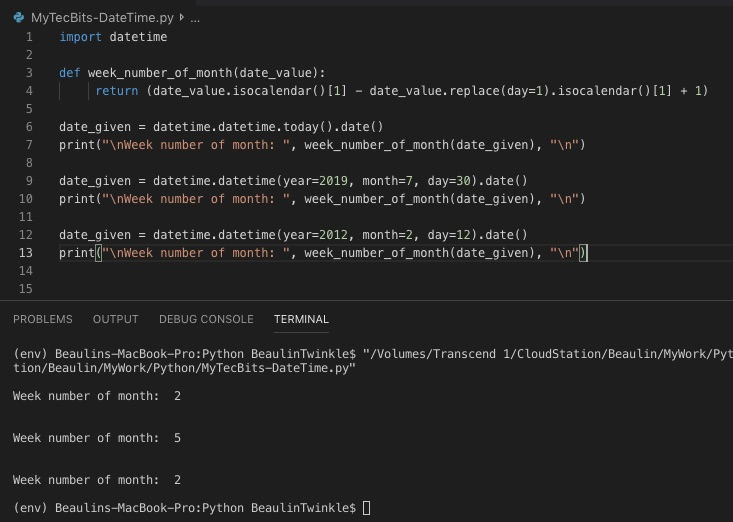# How to get week number of month in Python?

In my earlier python tip, we have seen how to get the week number of year using the date.isocalendar() method in the datetime module. This time, we will see how to get the week umber of month. However, finding the week number of a given date in a month is not a straightforward way. There is no inbuilt method to get this. We have to create our own logic using the existing modules and methods to get it. Here is one of the method I’m using to find the monthly week number in Python programming.

In this logic, I have used replace() method to get the first day of the month for the given date. Then used isocalendar() method to get the yearly week number for the given date and the first day of month. Finally subtracted the yearly week number of first day of the month from yearly week number of given date and added 1 to get the monthly week number of the given date.

## Example

```import datetime

def week_number_of_month(date_value):
return (date_value.isocalendar() - date_value.replace(day=1).isocalendar() + 1)

date_given = datetime.datetime.today().date()
print("\nWeek number of month: ", week_number_of_month(date_given), "\n")

date_given = datetime.datetime(year=2019, month=7, day=30).date()
print("\nWeek number of month: ", week_number_of_month(date_given), "\n")

date_given = datetime.datetime(year=2012, month=2, day=12).date()
print("\nWeek number of month: ", week_number_of_month(date_given), "\n")
```

## Reference

### 2 thoughts on “How to get week number of month in Python?”

1.Thank you, it’s what I was looking for.

Reply
2.in some special cases (e.g. date_given = datetime.datetime(year=2019, month=12, day=30).date()) returns negative values

Reply

This site uses Akismet to reduce spam. Learn how your comment data is processed.